This section is Section 2 of 3.

Speed as well as accuracy is important in this section. Work quickly, or you might not finish the paper. There are no  penalties for incorrect responses, only marks for correct answers, so you should attempt all 27 questions. Each question is worth one mark.

You must complete the answers within the time limit. Calculators are NOT permitted.

Good Luck!

Note – if press “End Exam” you can access full worked solutions for all past paper questions

Which of the following could result in a homeostatic response?

1. A rise in an internal condition.

2. A reduction in an internal condition.

3. A rise in an external condition.

4. A reduction in an external condition.

1.
0
0

## Explanation

All 4 Statements are correct. To maintain a constant internal environment, a rise and reduction in internal and external conditions can be counteracted by hormones, to restore the original balanced state. Although it may seem that external conditions should not be included in the answer, external conditions are not specified here – they can apply to external stimuli, which can be cause nervous responses, action of chemicals on the body (restricted to the skin), which can be counteracted by hormones etc.

Post Comment

2.74g of 1-bromobutane is reacted with excess aqueous sodium hydroxide to produce 1.11g of butan-1-ol according to the equation below.

C4H9Br + NaHO → C4H9OH + NaBr

(Ar : H = 1; C = 12; O = 16; Na = 23; Br = 80)

2. What is the percentage yield of butan-1-ol?
0
0

## Explanation

To calculate the theoretical yield, as percentage yield = actual/theoretical x 100, it must be noted that the mole ratio of 1- bromobutane : butan-1-ol is 1:1. Hence, calculate the moles of 1-bromobutane: n(1-bromobutane) = m/Mr = 2.74/ (4(12)+9(1)+80) = 0.02

0.02 is in the same moles as butan-1-ol. Hence, molar mass of butan-1-ol:

Mr (butan-1-ol): 4(12) + 9(1) + 16 + 1 = 74

Mass (butan-1-ol): 0.02 x 74 = 1.48

Hence, 1.11/1.48 x 100 = 75%

Ignore sodium at all costs – they have given its Ar to trick you. Sodium is in excess, and it is not the compound which partakes in either reacting species – 1- bromobutane nor butan-1-ol!

Post Comment

Nuclide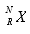is an unstable isotope which decays in two stages into nuclide Z as shown: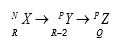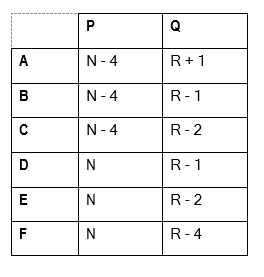3. What are the values of P and Q?
0
0

## Explanation

P = N-4, as this is alpha decay. This is as, the atomic (proton) number decreases by 2, and this is only possible via alpha decay. Alpha decay leads to a loss of 4 nucleons. As P is conserved in Z, there is no change in mass number. The only emission that conserves mass number , is beta (positive – in this case) decay. However, proton number decreases by 1, as a proton turns into a neutron, and releases a positron (antielectron).

Post Comment

The design in the diagram is formed from four circles which all touch at the top of the shape (the diagram is not to scale).The diameter of the smallest circle is d, the second is 2d, the third is 3d and the largest is 4d.

4. Find an expression for the area of the shading in the design.
0
0

## Explanation

Remember to answer in terms of diameter – although radius is required for the area, express r as, r = d/2.

Subtract the  2nd largest circle from the largest circle:

Largest Area: π(4d/2)²= 4π

2nd  Largest Area: π(3d/2)²=9/4π

Largest A –2nd Largest A => 4π – 9/4π = 7/4π

Subtract the smallest circle from the 3rd largest circle:

3rdLargest Area: π(2d/2)²= π

Smallest Area: π(d/2)²=1/4π

3rd Largest  Area – Smallest Area→ π– 1/4 π = 3/4π

Hence, adding these areas together: 3/4π+ 7/4π= 10/4π => 5/2πd²

Post CommentSam Medicmind Tutor

Tue, 02 Nov 2021 18:34:22

You must be fucking skunked to answer this

The diagrams below show part of the breathing apparatus of a human.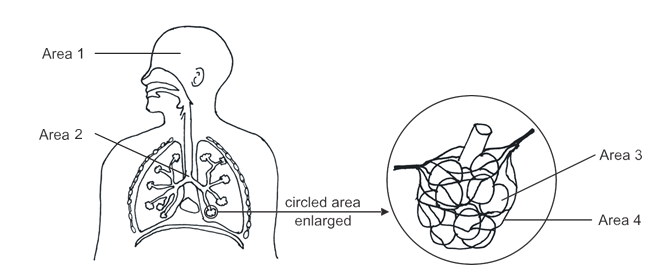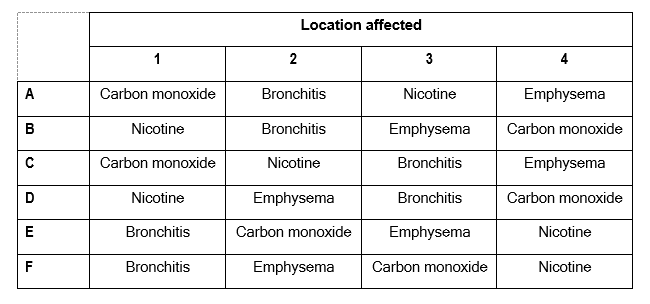5. Which row of the table correctly identifies the locations affected by two chemicals found in cigarettes and by two diseases associated with smoking?
0
0

## Explanation

Nicotine affects the brain as it creates a dependency for tobacco inhalation via smoking – addiction. It releases glucose from the liver, adrenaline from adrenal glands and various neurotransmitters in the brain, increasing the sensitivity of the brain’s reward system to rewarding stimuli.

Bronchitis: inflammation of the bronchi (airways) in the lungs. It can cause coughing, wheezing, shortness of breath etc., as the inflammation restricts air flow into the lungs, making it harder to breathe. The walls of the bronchi produce mucus to trap particles (in the case of smoking, this is tobacco smoke), leading to inflammation.

Emphysema: Due to frequent infection, phagocytes are attracted to the lungs where they release elastase – an enzyme which breaks down the elastin in the alveolar walls, to enable them to reach the surface where the bacteria are. Without adequate elastin, the alveoli cannot stretch, so they recoil and burst. This causes large air spaces, reducing the surface area for gaseous exchange, causing rapid breathing. Alveolar air sacs break down and fuse together, leading to severe breathing difficulties.

Carbon Monoxide: Carbon monoxide is released in tobacco smoke, and enters the blood vessels. Red blood cells have a higher affinity to carbon monoxide, in comparison with oxygen. Hence, more red blood cells bind irreversibly to carbon monoxide, rather than oxygen. Respiration cannot occur sufficiently for metabolic processes to occur, hence breathing becomes very short and rapid. Carbon monoxide poisoning is very critical as this directly affects respiration.

Post Comment

The diagram represents the molecule lecithin, a chemical that is added to chocolate drinks to prevent the oils separating out from water.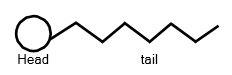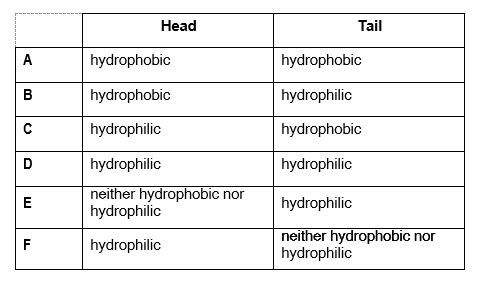6. Which row of the table below correctly identifies the characteristics of the molecule?
0
0

## Explanation

The head is hydrophobic, which repels water and ionic substances. The tail is hydrophilic, as it is attracted to water and ions soluble in water. This is as, the oils are larger than water in size, and so a large surface area, the hydrophobic head, attracts these oils. Water molecules, which are present in a higher quantity due to their size, cluster around the tail in abundance. This forms an emulsion of oil and water, as the molecule is classified as an emulsifier.

Post CommentSam Medicmind Tutor

Tue, 02 Nov 2021 18:35:34

Whoever made this question needs their pussy ate, bon apple teeth.

Students investigate a radioactive They place a detector close to a radioactive source and take 5 readings over 5 minutes. They then place a thin sheet of paper between the detector and the source, and again observe the counts over 5 minutes. Lastly they replace the paper sheet with an aluminium one and observe the counts over 5 minutes. Their results are shown below:

 Nothing Paper Aluminium Reading 1 100 101 30 Reading 2 98 102 31 Reading 3 99 96 28 Reading 4 103 101 33 Reading 5 101 103 27
7. What type(s) of radiation is being given off by the source?
0
0

## Explanation

It is definitely not alpha, as alpha is stopped by a piece of paper, and it has a very short range in air, and would be very minimally detected. Beta and gamma pass through paper, however beta is stopped by aluminium. Hence, in aluminium, it is almost only gamma rays.

Post Comment

A formula which connects the ‘going’, G, with the ‘rise’, R, of stairs in a staircase is below: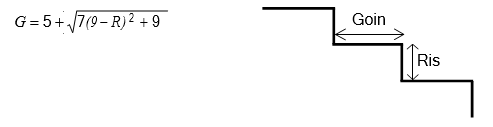8. Rearrange the formula to give R in terms of G.
0
0

## Explanation

Firstly, subtract 5 from both sides.

G – 5 = √(7 (9-R)²+9)

Square both sides:(G – 5)² = 7(9-R)²+9

Subtract 9 on both sides: (G – 5)²– 9 = 7(9-R)²

Divide by 7 on both sides: ((G – 5)²– 9) / 7 = (9-R)²

Square root both sides: √(((G – 5)²– 9) / 7) = 9-R

Add R to both sides and subtract √(((G – 5)²– 9) / 7) on both sides:

R = 9 – √(((G – 5)²– 9) / 7)

Post Comment

In one type of neurological disease, neurons are unable to detect a certain stimulus which would normally cause pain. In a patient with this disease, which of the following would be true?

1. The patient would not show a reflex action to the stimulus.

2. The patient would be able to take appropriate action if they could also see the application of the stimulus.

3. The patient would be able to sense pain but would not be able to respond to it.

4. The patient would be able to sense pain but would not be able to communicate this to the brain.

9.
0
0

## Explanation

Only Statements 1 and 2 are correct. A reflex action would not occur, as neurons (which are used in the reflex arc to give a response) cannot detect the stimulus. As the stimulus is not detected, an electrical impulse cannot travel to other neurons, through synapses, to give an appropriate reflex response. However, if the stimulus is seen (i.e. fire), despite not experiencing it, one can withdraw one’s limbs from it. Statements 3 and 4 are incorrect. This is as, the stimulus can’t even be interpreted as pain, as the neurons which can cause pain detection, are not functioning. Without the sensation, action cannot be taken. Statement 4 is contradictory – pain is interpreted by the brain, hence the brain’s involvement is key in detection. The patient is only aware of pain after the brain interpretation.

Post Comment

Inorganic builders are put into detergents to act as a buffer, e. to keep the pH close to 7. Sodium tripolyphosphate is a major one used. The equation for its formation is shown below:

aNaH₂PO₄ + bNa₂HPO₄ → cNa₅P₃O₁o + dH₂O

10. What are the values of a, b, c and d?
0
0

## Explanation

LHS: Na = 3, H = 3, P = 2, O = 8. RHS: Na = 5, P = 3, O = 11, H = 2

Firstly, considering Na: if b=2, Na is doubled on LHS giving 2Na2HPO4 ->

LHS: Na = 5, H =4, P = 3, O = 12 (Na is 5 on both sides)

Hence, as H = 4, let d = 2 on RHS: 2H2O RHS: Na = 5, P= 3, O = 12, H =4

Both sides are balanced, hence a = 1, c=1.

NaH2PO4 + 2Na2HPO4 Na5P3O10 + 2H2O

Post Comment

The diagrams show, not to scale, three different situations in which a force F acts. Also shown in each case is a distance d.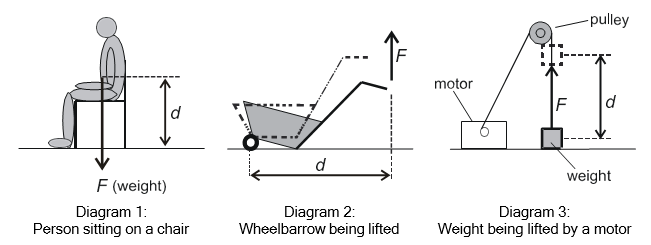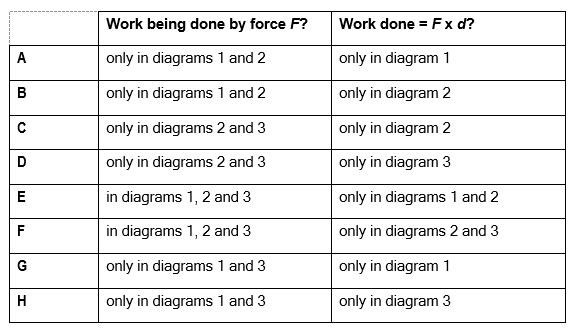11. Which line in the table shows whether or not work is being done by force F in each situation and, if so, whether the work done is equal to F x d?
0
0

## Explanation

In diagrams 2 and 3, work is being done by force F, however, this occurs at a component of d at an angle θ in Diagram 2. This is as, the work is not being done in the direction of D, yet it is done at an angle in the direction of d. Hence, an appropriate formula would be: W = Fdcosθ

In Diagram 3, work is being done in the direction of the Force and the vertical distance, as the pulley is pulling the weight vertically up, through a vertical distance of f. Diagram 1 – no work is being done by F, as the force is opposed by the resistance of the chair – the force is not actively moving the object in that distance, the object is stationary – it is not a vector due to this stationary nature.

Post Comment

Evaluate: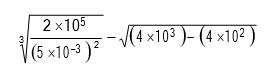12.
0
0

## Explanation

Here, for the first power, there is a lot of cancelling – it can be re-written as: ((2 X  105) / (25 X 10-6))1/3 = (2/25 X 1011)1/3= (8 X 1011)1/3= 2000

For the second power, convert it all to the smallest power – 10240X102– 4X102= 36 X 102

√(36 X 102) = 6X10 = 60

Hence, 2000-60=1940

Post Comment

The left diagram shows a plate containing a jelly with one species of bacterium evenly spread through Placed on the jelly are three discs called P, Q and R. They have been treated as in the key.

The right diagram shows the plate 48 hours later.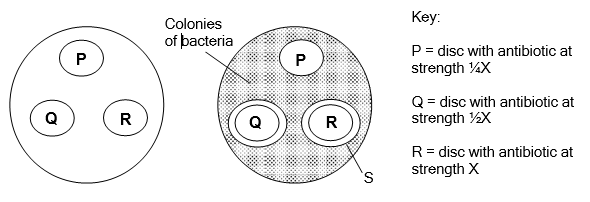1. Antibiotic at strengths X and ½X are equally as effective.

2. Bacterial resistance to this antibiotic occurs at all three strengths.

3. S may represent the maximum distance the antibiotic has diffused out of the disc.

13. Which of the following is/are possible explanations for the result?
0
0

## Explanation

Statement 1 is correct – this is as, the distance between the circumference of the antibiotic and the circumference of region S, which can be defined as the region in which there are no bacterial colonies, is the same in both antibiotics. Hence, both antibiotics are equally as effective, if they restrict an equal amount of growth.

Statement 2 is incorrect and too vague – it is unknown whether antibiotic resistance has spread across the population, as the same antibiotic, in different strengths, produces the same results. From observation, it only seems that the bacteria are resistant to antibiotic P, however, the assumption of resistance would be incorrect, as the antibiotics are the same – their strengths only differs.

Statement 3 is correct – S may represent the maximum distance the antibiotic has diffused out of the disc, hence restricting bacterial growth up to a certain point in the plate.

Post Comment

Azurite is a mineral made up of a mixture of CuCO3 and Cu(OH)2.

14. Which one of the following is a possible formula of azurite?
0
0

## Explanation

CuCO3 + Cu(OH)2 ?

Firstly, write down the quantities of each element on the LHS:

Cu = 2, C = 1, O = 5, H =2. As O =5, it cannot be A as this only has 4 O. This ratio is insufficient to answer the question.

That means that, a coefficient of 2 must be added to either CuCO₃ or Cu(OH)₂. If the 2 is on Cu(OH)₂, the answer can be E or F only, as the number of O = 7 now, excluding all other answers. Hence, let’s try to add 2 to CuCO₃.

2CuCO₃ hence, Cu = 2, C = 2, O = 6

Combining with Cu(OH)₂, Cu = 1, O = 2 and H=2

The final balanced equation is: 2CuCO₃ + Cu(OH)₂ Cu₃C₂H₂O₈

Post Comment

The microwaves generated in a microwave oven travel through air at a speed of 3.0 x 108m/s, with a wavelength of 12cm. They pass through plastic food containers, but at a reduced speed of 2.0 x 108m/s.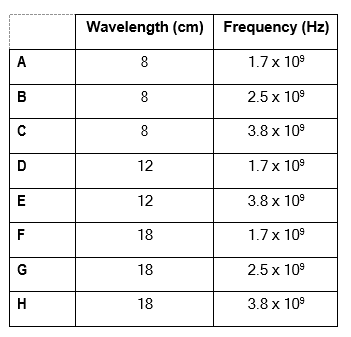15. What are the wavelength and frequency of these microwaves as they pass through a plastic food container?
0
0

## Explanation

Frequency is invariant, hence use the speed in a vacuum to calculate it: c=fλ ; f = c/λ (3 x 108)/(12 x 10-2) = 2.5 x 109 λ = c/f (using new speed): (2 x 108)/( 2.5 x 109) = 0.08 m = 8 cm

Post Comment

In the triangle ABC shown below (not to scale):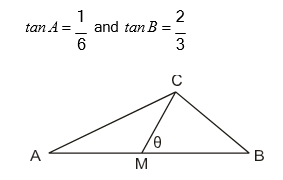M is the midpoint of AB.

16. What is the value of tanӨ
0
0

## Explanation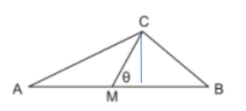To find tan θ, a vertical line must be drawn from C to the line AB, as an opposite and adjacent side, with respect to a right angled triangle, is needed. This line also maps out the triangle CAD (where D is the vertex of the triangle of angle A, and of the triangle of angle θ). This line also gives the triangle CBD, corresponding to the angle at B. tan A = CD/AD = 1/6 Hence, CD (according to A) = 1, AD=6 (according to A)

Tan B = CD/BD = 2/3 Hence, CD = 2, BD = 3 – the tan of A is given at a scale factor of 2. Multiplying the tan A by 2 to standardise both fractions – tan A = 2/12. Hence,

CD = 2, AD = 12. As M is the midpoint of AB, AB = AD + DB = 12 + 3 = 15.

AM = 15/2. For tan θ = CD/MD

Hence, MD = 12-7.5 = 4.5

Tan θ = 2/4.5 = 4/9

Post Comment

Consumption of alcohol leads to an increase in dilute urine production by reducing the level of the hormone ADH.

1. ADH travels in the bloodstream.

2. There is a positive correlation between the level of ADH and the production of dilute urine.

17. Which of the following are correct statements about alcohol and/or ADH?
0
0

## Explanation

Statements 1 and 4 are correct. ADH is a hormone, hence it travels through the bloodstream. A reduction in ADH causes less volume of water reabsorbed by the kidneys; if ADH successively decreases, there is a successive decrease in the volume of water reabsorbed, thus leading to dehydration. Statement 2 and 3 are both incorrect – there is a negative correlation between dilute urine production and level of ADH. This is as, a greater release of ADH causes more water reabsorption in the blood, leading to more concentrated urine. Less release of ADH causes less water reabsorption, leading to more dilute urine.

Post Comment

Vanadium is a metal that lies just above zinc in the reactivity series.

18. Which one of the following could not be used to obtain the metal from its ore?
0
0

## Explanation

As vanadium lies above zinc, zinc lies directly above iron. Hence, iron cannot displace vanadium sulfate to obtain vanadium on its own.

Post Comment

The circuit shows three lamps and two ammeters in a circuit.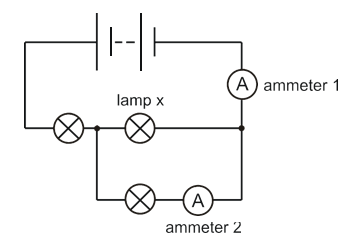Lamp X ‘blows’ (the filament breaks).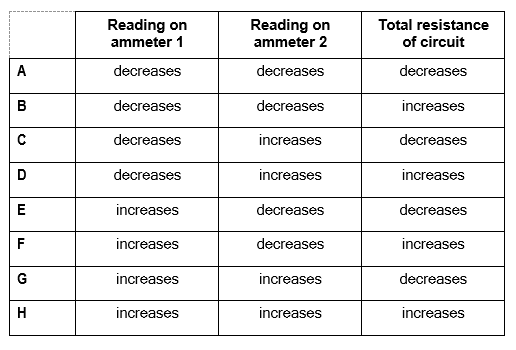19. What happens to the reading on each ammeter, and to the total resistance of the circuit?
0
0

## Explanation

Reading on ammeter 1 decreases, as the current cannot pass through the broken lamp, hence the current being received is from the parallel branch. Reading on ammeter 2 increases, as more current flows through this branch, due to having less resistance – the broken lamp provides resistance for the current, as it’s harder for charge carriers to pass through a non- functional conductor. The total resistance of the circuit increases, as the broken lamp acts as a ‘resistor’, as it opposes the movement of charge carriers.

Post Comment

In a fairground game there are two bags, each of which contains 4 coloured balls. There are a total of 4 red balls, 3 yellow balls and 1 blue ball. The player chooses one of the bags and removes two balls without replacing them. If the two balls are the same colour then the player wins. The player is equally likely to choose either bag and the balls are arranged to give the smallest possible probability for the player to win.

20. What is the probability that the player wins?
0
0

## Explanation

The smallest probability to win is if you split the 4 red balls so that two are in each bag, and then 1 bag will consist of 2 yellow and 2 red, and the other bag will consist of 1 yellow, 1 blue and 2 red.

There are three ways that you can win

Pick bag 1 and then get 2 yellow

Pick bag 1 and then get 2 red

Pick bag 2 and then get 2 red

P(bag 1 and 2 yellow) = P(Bag 1) X P(first yellow) X P( 2nd = yellow)

P(Bag 1) = 1/2,

P(first = yellow) = 2/4, i.e. two yellows in a bag that contains 4 balls

Once this is removed, only 3 balls remain in the bag and only 1 is yellow. Given that the first was yellow,

P(second is also yellow) = 1/3

P(bag1 and 2 yellows) = 1/2 X 2/4 X 1/3 = 2/24 = 1/12

P(Bag 1 and 2 reds) = 1/2 X 2/4 X 1/3 = 1/12

P(Bag 2 and 2 reds) = 1/2 X 2/4 X 1/3 = 1/12

Hence, the probability of winning = 1/12 + 1/12 + 1/12 = 3/12 = 1/4

Post Comment

In a monohybrid genetic cross a ratio of phenotypes of 3:0 rather than the usual 3:1 ratio was seen. This could be due to…

1. offspring with both dominant alleles not

2. only a small number of offspring being produced.

3. chance

21. This could be due to:
1
0

## Explanation

Statement 1 is too vague, as it doesn’t specify whether the offspring die within the womb or after birth. If the offspring die within the womb, the alleles of the offspring cannot be known, to deduce it to be dominant.

However, if there is a method of knowing these alleles, they are to be excluded. If the offspring die after birth, due to dominant alleles, this ratio is still included as it is post-birth death. Due to this ambiguity, it cannot be Statement 1. The ratio 3:0 could be obtained, if there are a small number of offspring produced (i.e. only 3 offspring produced, with all 3 phenotypes). This can be the case, as we are not given the number of offspring. As ratios can be amplified, i.e. 3:1 = 6:2 etc, it may be due to a matter of chance, that a population of offspring repeatedly express one phenotype; fertilisation is a random process, hence outcome is only probabilistic.

Post Comment

Tritium, symbol T, is hydrogen with a mass number of 3. It is radioactive and undergoes β decay.

 1 HeOH 2 H2O, O2 and He 3 H2O, H2 and He
22. Which of the following could be the only product(s) after a quantity of HTO undergoes decay?
0
0

## Explanation

If hydrogen undergoes Beta decay, a neutron turns into a proton and the mass number is not changed – still 3. HTO – 1 hydrogen, 1 tritium and 1 oxygen. Hydrogen has atomic number 1, so it has 1 proton in its nucleus.

Helium has atomic number 2, so it has 2 protons in its nucleus.Tritium is an isotope of hydrogen, so it contains 1 proton.. Since tritium’s mass number is 3, a tritium nucleus must also contain 2 neutrons. Hence, tritium is 1 proton and 2 neutrons.

Beta minus decay turns a neutron to a proton (and releases an electron and antineutrino). So after beta minus decay of tritium, the remaining nucleus contains 2 protons and 1 neutron. Hence, it’s now an isotope of helium: He – 3

Hence, tritium has turned to helium. T→He

HTO therefore initially becomes HeOH.

But He is a noble (inert) gas, so it is chemically unreactive and does not bind to oxygen or hydrogen

Therefore HeOH OH + HE

Since there is initially ‘a quantity’ of HTO, a large amount of OH is produced. The OHs will react together as follows:

4OH → 2H₂O+ O₂

So we are left with H₂O, O and He, corresponding to answer choice 2 only.

Post Comment

A cyclist and a bike have a combined mass of 100kg. The cyclist free-wheels (rolls without pedalling) at a constant speed of 0.8m/s down a 1 in 10 slope (this means that the cyclist descends 1.0m for each 10m travelled along the road, as shown in the diagram.)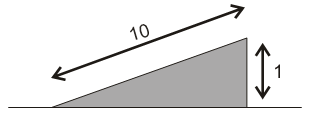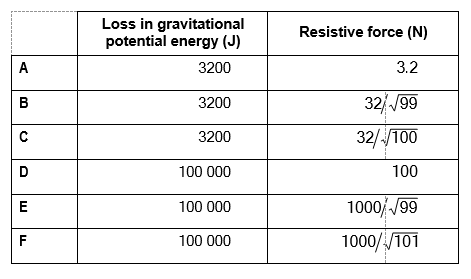23. Calculate the loss in gravitational potential energy as he loses 100m in vertical height and hence calculate the total resistive force on the cyclist. [g = 10N/kg]
0
0

## Explanation

Loss in gravitational potential = mgh = 100 x 10 x 100 = 100 000J. At constant speed, the resistive force = weight component parallel to incline

Hence, F = mgH/L

L = incline = 10m

H = change in horizontal = 1m

100 x 10 x 1/10 = 100N

Post Comment

The new sign for a local business contains two different One of the sections will be produced from wood, while the other will be metal. Metal is three times as expensive as wood. The cost of metal needed for each sign is proportional to the diameter of the sign, while the cost of wood needed is proportional to the square of the diameter. If the diameter of the sign is doubled, then the total cost of the materials will be tripled.

24. What percentage (to the nearest 1%) of the sign is metal?
0
0

## Explanation

Units of wood with price x/unit => cost ax

b units of metal with price 3x/unit => cost 3bx

But ax =

k1X D , where D = diameter

and 3bx = k2 X D2

Total cost: x(a + 3b) =k1D+k2D2= D(k1+k2D) ,

Double diameter = triple to cost 3x(a + 3b)=k12D+k22D2= 2D(k1+k22D)

Divide both equations: 3 = 2(k1+k22D) / (k1+k2D)

3k1+4k2D

k1= k2D

k2/k1= 1/D

From above, ax =k1D

3bx = k2D2

Divide the equations

3b/a =k2/k1D

b/a = 1/3 (k2/k1D) = 1/3 (1/(D X D)) = 1/3

Part of the metal: b/(a+b) = (b/a) / (1 + b/a) = (1/3) / (1 + 1/3) = 1/4 = 25%

The proportion of metal is 25%

Post Comment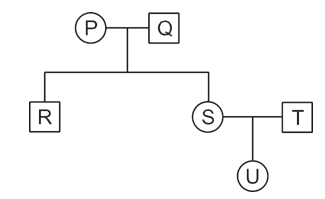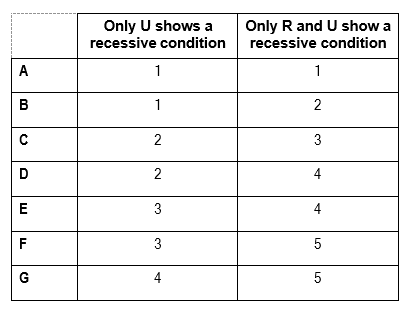25. What is the minimum number of people shown in the family pedigree who must be heterozygous for the two situations described in the table in the absence of any new mutations?
0
0

## Explanation

For a recessive condition to be expressed, the individual must contain 2 recessive alleles. The question asks for the minimum number of heterozygous individuals required for a recessive condition to be expressed.

Let D = dominant, d = recessive

For U to only be recessive: The parents (S and T) both are required to be Dd and Dd.

 D d D DD Dd d Dd dd

As we require the minimum heterozygous number, the parents of S produce 1 heterozygous dominant (Dd) and one homozygous dominant R (DD). Hence, P and R have to be: DD and Dd for this to occur, with either P or R having either genotypes.

 D d D DD Dd D DD Dd

Hence, the parents of U: S and T are both Dd, and either P or R (U’s grandparents) are Dd. Therefore, the minimum number of heterozygous for this situation is: 3.

For R and U to only be recessive: We have already established that for U to be recessive, S, T and either P or Q are heterozygous. For R to be recessive too, we need to eliminate the homozygous dominant, and replace it with heterozygous dominant. Hence, both P and Q are Dd (heterozygous dominant), as with the case of S and T.

 D d D DD Dd d Dd dd

The parents of U: S and T are both Dd, and the parents of R are both Dd, totaling 4 heterozygous dominant alleles.

Post Comment

A mixture of C2H4(g) and H2(g) is placed in a sealed container. Some finely divided nickel is added as a catalyst to the The temperature rises to 150°C and the two gases slowly react to give C2H6(g).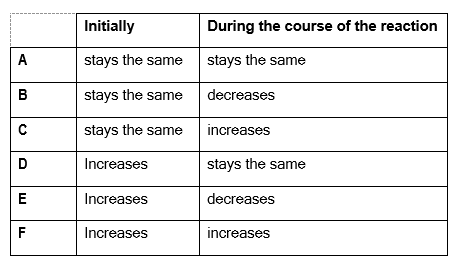26. Which row of the table correctly identifies how the pressure changes after the nickel is added?
0
0

## Explanation

The pressure must decrease during the course of the reaction because looking at the equation for the reaction, we can see that there would be two moles of reactant gases per one mole of gaseous product. This means that, assuming the gases are ideal so one mole of any of the gases occupies the same volume, the pressure will decrease as more product is formed and less reactant remains as the reaction proceeds, due to a decrease in the number of moles of gas present. So the answer must be B or E.

Because the reaction is taking place in a sealed container, adding any volume of substance initially to the 2 reactant gases would decrease the volume available for the gases to occupy, causing a resultant increase in pressure of the gases. This is the effect the nickel added has initially, so the answer is E.

Post Comment

A sound wave is produced by a loudspeaker cone, which creates pulses of pressure by moving back and forth between two points X and Y as shown in the diagram.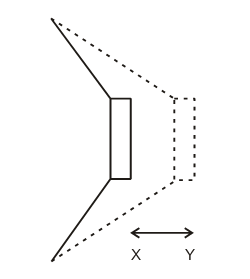The distance between points X and Y is 5.0mm and the loudspeaker produces pulses of pressure every 0.2 milliseconds.

P It has a speed of 25m/s

Q It has an amplitude of 5.0mm

R It has a wavelength of 5mm

S It has a fundamental frequency of 0kHz

27. Which of these statements can be correctly deduced from the information given?
0
0

## Explanation

Only the distance and time period are given. Hence, amplitude and wavelength cannot be determined. The frequency can, as we are given the time period (pulses of pressure every 0.2 milliseconds). F = 1/T. Hence, 1/(0.2 x 10-3) = 5000 Hz = 5.0 KHz

Post CommentDebbie Medicmind Tutor

Mon, 25 Oct 2021 11:44:52

S is written wrong as it says 0KHz for the frequency when it should say 5KHZ.

## Instructions

Below is a summary of your answers. You can review your questions in three (3) different ways.

The buttons in the lower right-hand corner correspond to these choices:

2. Review questions that are incomplete.
3. Review questions that are flagged for review. (Click the 'flag' icon to change the flag for review status.)

You may also click on a question number to link directly to its location in the exam.

## Instructions

This review section allows you to view the answers you made and see whether they were correct or not. Each question accessed from this screen has an 'Explain Answer' button in the top left hand side. By clicking on this you will obtain an explanation as to the correct answer.

At the bottom of this screen you can choose to 'Review All' answers, 'Review Incorrect' answers or 'Review Flagged' answers. Alternatively you can go to specific questions by opening up any of the sub-tests below.

x

## When should we call you?

It would be great to have a 15m chat to discuss a personalised plan and answer any questions

## What time works best for you? (UK Time)

Pick a time-slot that works best for you ?

Submit

Submit